# Squares & Square Roots

## Squares & square Roots of Class 7

We have already studied that the square of a number is the product of the number with the number itself. For example a given number is x, the square of x is (x x x), denoted by x2.

e.g. (9)2 = 9 x 9 = 81

(5)2 = 5x 5 = 25

(10)2 = 10x 10 = 100

(1.2)2 = 1.2x 1.2 = 1.44

### Perfect Squares or Square Numbers

A natural number is called a perfect square or a square number if it is the square of some natural numbers. It is always expressible as the product of equal factors.

e.g.

49 = 7x 7 = 72

144 =2 x 2 x 2 x 2 x 3 x 3 = 12x 12 = 122

225 = 5x 5x 3 x 3 = 15x 15 = 152

1000= 2 x 2 x 5x 5 = 10x 10 = 102

Example. Show that 500 is not a perfect square.

Ans. Resolving 500 in to prime factors, we get

500 = 2 x 2x 5x5 x 5

Making pairs of equal factors, we find that 5 is unpaired

Hence 500 is not a perfect square.

### Properties of Perfect Squares

Example 1. A number ending in 2, 3, 7 or 8 is never a perfect square

e.g. 62,103,113,148 ,237,107.

Example 2.  A number ending in an odd number of zeros is never a perfect square.

e.g. 10,3000 , 13000, 120, 100000.

Example 3. The square of a even number is always even.

e.g.122 = 144, 162= 256 302 = 900 etc.

Example 4. The square of an odd number is always odd.

E.g.52 = 25, 72 = 49, 132 =169, 112 = 121 etc.

Example 5. The square of a proper fraction is smaller than the fraction.

e.g.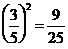and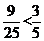since 9x 5 < 25x 3.

Example 6. For every natural number n we have

(n + 1)2– n2 = (n + 1 + n) (n + 1 – n) = {(n + 1) + n}

{(25)2– (24)2} = (25 + 24) = 49

{(70)2– (69)2} = (70 + 69) = 139

Example 7. For every natural number n, we have sum of the first n odd natural numbers = n2

E.g. {1+3+5+7+9+11+13}=Sum of first 6 odd numbers=62=36

{1+3+5+7+9+11+13+15+17+19}}=Sum of first 10 odd numbers=102=100

### Pythagorus Triplets

A triplet (m, n, p) of three natural number. m, n and p is called a Pythagoras triplet of m2 + n2 = p2

E.g. (3, 4, 5) (5, 12, 13), (8, 15, 17), etc.

Example 8. For every natural number m greater than 1, (2m, m2– 1, m2 + 1) is a Pythagoras triplet.

Find the Pythagoras triplet where smallest number is 10.

Ans. For every natural number m > 1, (2m, m2– 1, m2 + 1) is a Pythagoras triplet

For every natural number m > 1, (2m, m2– 1, m2 + 1) is a Pythagoras triplet.

Put 2m = 12 m = 6

m2– 1 = 35

m2 + 1 = 36

triplet (12, 35, 37)

### Some Interesting Patterns of Square Numbers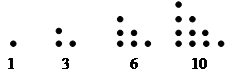Sum of first two is 4

Sum of second and third is 9

Sum of third and forth is 16… and so on

Thus nth triangular number is given by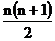Part I. There are 2n non-perfect square numbers between two consecutive square number n2 and (n + 1)2.

e.g. Between the number 52and42.Total 8 numbers are between these two numbers.

Between the number 502and492.Total 98 numbers are between these two numbers.

Part II. The sum of first n odd natural numbers in n2

n = 1 1 = 1 = 12

n = 2 1 + 3 = 4 = 22

n = 3 1 + 3 + 5 = 9 = 32

n = 4 1 + 3 + 5 + 7 = 16 = 42

n = 5 1 + 3 + 5 + 7+9 = 25 = 52

n=10 1+3+5+7+9+11+13+15+17+19 =102

Part III. If 1 is added to the product of two consecutive odd natural numbers, it is equal to the square of the only even natural number between them.

In general

(2n – 1) x (2n + 1) + 1 = 4n2 = (2n)2

this pattern is very useful to find the result

1 x 3 + 1 = 4 = 22

3 x 5 + 1 = 16 = 42

5 x 7 + 1 = 36 = 62

7 x 9 + 1 = 64 = 82 etc.

In reverse way we can factorize (2n)2-1 in two factors as (2n – 1) x (2n + 1)

Part IV. If 1 is added to the product of two consecutive even natural numbers, it is equal to the square of the only odd natural number between them.

In general

2n (2n + 2) + 1 = 4n2 + 4n + 1 = (2n + 1)2

E.g

2 x 4 + 1 = 9 = 32

4 x 6 + 1 = 25 = 52

6 x 8 + 1 = 49 = 72

8 x 10 + 1 = 81 = 92

10x 12 + 1 = 121 = 112

12x 14 + 1 = 169 = 132

and so on ………………

In reverse way we can factorize (2n + 1)2-1 in two factors as 2n (2n + 2)= 4n(n+1)

Part V. Squares of natural numbers having all digits 1 follow the following pattern.

112 = 121

1112 = 12321

11112 = 1234321

111112 = 123454321

1111112 = 12345654321

and so on …………

we can use this in many ways as

222 = 4 x121=484

3332 = 9 x12321 =110889

2222222 = 4 x12345654321= 49382737284

Using suitable patterns, compute the following

(i)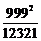(ii)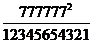Ans. We have the following pattern

112 = 121

1112 = 12321

11112 = 1234321

111112 = 123454321

1111112 = 12345654321

(i)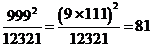= 81.

(ii)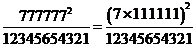= 49.

Part VI. Observe the following pattern

72 = 49

672 = 4489

6672 = 444889

66672 = 4444889

666672 = 44444889

and so on

### Some Short–Cuts to Find Squares

Three column method – This method is based upon an old Indian method of multiplying two numbers. It is convenient for finding squares of two digit numbers only. This method uses the identity (a + b)2 = a2 + 2ab + b2

Step I : Make three columns and write the value of a2, 2 x a x b and b2 respectively in these columns as follows:

As an illustration let us take ab = 34 = 3x10+4

a = 3 and b = 4

 Column-I Column-II Column-III a2   9 2 x a x b   24 b2   162222

Step II : Undertake the units digit of b2 ( in column III) and add its tens digit, if any, to 2 x a x b (n column II)

 Column-I Column-II Column-III 9 24   +1 25 162222

Step III : Undertake the units digit in column II and add the number forward by tens and other digit, if any to a2 in column II.

 Column-I   a2 Column-II   2 x a x b Column-III   b2 9   +2 24   +1 162222 11 25

Step IV : Under the number in column I

 Column-I   a2 Column-II   2 x a x b Column-III   b2 9   +2 11 24   +1 25 162222 11 5 6

Step V: Write the understual digits at the bottom of each column to obtain the square of the given number.

In this case, we have 342 = 1156.

### Some Particular Methods

Rule1: The square of a number of the form a 5 (where a is tens digit and 5 is units digit) is the number which each in 25 and has the number a(a + 1) before 25.

Example: Find the squares of the following numbers

1. 45
2. 95
3. 85

Ans. 1. Here we have

a = 4=> a(a + 1) = 4× 5 = 20

(45)2 = 2025

2. Here, we have

a = 9 => a(a + 1) = 9 x 10 = 90

\952 = 9025.

3. Here, we have

a = 8=> a(a + 1) = 8x 9 = 72

752 = 7225.

Rule 2 : The square of a number of the form ( 5x 10 + a ) (where a is units digit and 5 is ten digit) is equal to

(25 + a) x 100 + a2

(5 a)2 = (25 + a) x 100 + a2

Example: Find the squares of the following numbers

1. 51
2. 53
3. 56

Ans. 1. Here, we have

a = 1

(51)2 = (25 + 1) x 100 + 12 = 2600 + 01 = 2601.

2. Here, we have

a = 3

(53)2 = (25 + 3) x 100 + 32 = 2800 + 09

= 2809

3. Here, we have

a = 6

(56)2 = (25 + 6) x 100 + 62 = 3100 + 36 = 3136.

Rule 3 : The square of a three digit number (5x 100+ ax10 +b) (where a,b are integers from 0 to 9.

(5x 100+ ax10 +b) 2 = (250 + ab) x 1000 + (ax10 + b)2

Example: Find the squares of the following numbers

1. 516
2. 513
3. 504

Ans. 1. Given number is 516

a = 1, b= 6

(516)2 = (250 + 16) x 1000 + (16)2

= 266000 + 256

= 266256.

2. Here, we have

a = 1, b = 3

(513)2 = (250 + 13) x 1000 + (13)2

= 263000 + 169

= 263169.

3. Here, we have

a = 1, b = 3

(504)2 = (250 + 04) x 1000 + (04)2

= 254000 + 16

= 254016.

Rule 4 : The square of a number abc…..5 (i.e., a number having 5 at units place is obtained by affixing 25 to we right of the number n(n + 1), where n = abc….

Example: Find the square of the following numbers

1. 225
2. 265
3. 1235

Ans. 1. 225

Here n = 22

n(n + 1) = 22 x 23

= 506

Hence 2252 = 50625

2. 265

Here n = 26

n(n + 1) =26x 27

= 702

Hence 2252 = 70225

3. 1235

Here n = 123

(n + 1) = 123 x 124

= 15252

Hence 12352 = 1525225

### Related Pages

To score More in your class 7 refer NCERT Solutions for Class 7 Maths